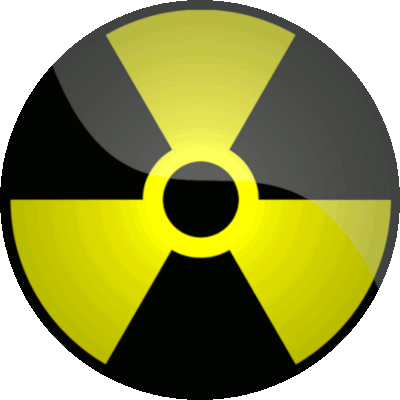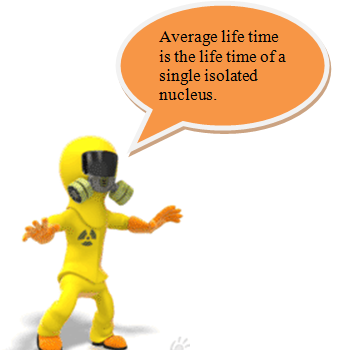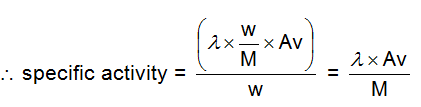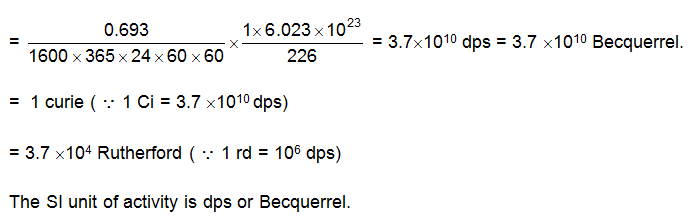Click to Chat

1800-1023-196

+91-120-4616500

CART 0

• 0

MY CART (5)

Use Coupon: CART20 and get 20% off on all online Study Material

ITEM
DETAILS
MRP
DISCOUNT
FINAL PRICE
Total Price: Rs.

There are no items in this cart.
Continue ShoppingRadioactivity

Table of Content

Half – Life

Average – Life Time

Activity

Specific Activity

Units of Activity

Related ResourcesAll radioactive decay follow 1st order kinetics and this is where the similarity ends.​

We measured the rate of reaction in chemical kinetics based on the rate of change of concentration of reactants or products.

But this procedure will not work for calculating the rate of radioactive reaction.

This is because most of the time the radioactive substance is a solid.

Therefore its concentration would be a constant with time (assuming it to be pure and that the product does not remain with the reactants).

Therefore the rate of radioactive reactions are measured by calculating the rate of change of nuclei of the radioactive substance. For a radioactive decay A ®B, the rate of reaction is calculated asWhere  =  decay constant of reaction

NA  = number of nuclei of the radioactive substance at the time when rate is calculated.

As you can see the above rate law is very much similar to the rate law of a first order chemical reaction, but all  other similarities ceases here.

For example unlike a chemical reaction the decay constant () does not depend on temperature. Arrhenius equation is not valid for radioactive decay.

Integrating the differential rate law we get

Where

No = number of  nuclei of A at t = 0

Nt = number of nuclei of A at t = t

=  decay constant

The expression can be rearranged to  give

Nt = Noe-lt                                          ... (1)

This suggests that the number of nuclei of radioactive substance A at any instant of time can be calculated, by knowing the number of nuclei at t = 0, its decay constant and the time.

Half – Life

Just like a 1st order reaction the half life of radioactive decay is given  by

t1/2 = 0.069/

Note

Let  us start with  10 nuclei. If the half life is 5 minutes, then at the end of first 5 minutes, number of nuclei  would be 5.
Now what would be the number of nuclei after next 5 minutes?
Will it be 2.5 or 2 or 3? We can clearly see that it cannot be 2.5 and if it is 2 or 3 then it cannot be called as half life. This dilemma can be overcome by understanding that all formula relating to kinetics are only valid when the sample size is very large and in such a large sample size ,a small difference of 0.5 will be insignificant.

The fact that radioactive decay follows the exponential law implies that this phenomenon is statistical in nature.

Every nucleus in a sample of a radionuclide has a certain probability of decaying, but there is no way to know in advance which nuclei will actually decay in a particular time  span.

If the sample is large enough – that is, if many nuclei are present – the actual fraction of it that decays  in a certain time span will be very close to the probability for any individual nucleus to decay.

To say that, a certain radioisotope has a half –life of 5 hr., then, signifies that every nucleus of this isotope has a 50  percent chance of decaying in every 5 hr. period.

This does not mean a 100 percent  probability of decaying in 10 hr. A nucleus does not have a memory, and its decay probability per unit time is constant until  it actually does decay. A half life of 5 hr. implies a 75% probability of decay in 10 hr., which increases to 87.5% in 15 hr, to  93.75% in 20 hr, and so on, because in every 5 hr.  interval the probability of decay is 50 percentAverage – Life Time

Average life time is defined as the life time of a single isolated nucleus. Let us imagine a single nucleus which decays in 1 second.

Assuming 1 second time interval to be very small the rate of change of nuclei  would be 1/1 (because –dN = 1 and dt =1).

We can also see that since

,

for a single isolated nucleus N = 1,

.

Therefore in this present case =1.

Now let us assume the same nucleus decays in 2 seconds, we can see that

i.e.,  is equal to ½. You will also notice that in the 1st case the nucleus survived for 1 second and in the second case it survived for 2 second. Therefore the life time of a single isolated

nucleus is  1/.  hence,  tav =

Activity

Activity by definition is the rate of decay  of a radioactive element. It is represented as ‘A’ and is equal to N.

By no means should activity be confused with rate of change of radioactive nuclei represented by
.

This is because  talks about the overall change in the number of nuclei in a given instant of time while activity only talks about that change which is decay.

If you go out to a market with Rs. 50 in your pocket and you spend Rs.20 in 5 minutes then your rate of change of money in the wallet is Rs. 4 / min and in fact the rate of spending the money is also Rs.4 / min. Here you can see both are same. But if while spending Rs. 20 in 5 minutes, somebody keeps   Rs.10 in your  wallet, then the rate of change of money in your wallet would become Rs. 2.5 /min, while the rate of spending the money is Rs. 4 / min.

This implies that as long as the radioactive substance is only decaying the rate of  change of nuclei and activity are same and equation () in terms activity of a radioactive substance can be written as
At =Ao e–t.

But if the radioactive substance is also being produced this  = rate of production – activity, (Of course it’s a different  matter, rate of production may or may not be a constant).

Specific Activity

This is activity per unit mass of the sample. Let radioactive sample weighing w gms have a decay constant .

The number of nuclei in the w gms would be (M/W)AV,

where M = molecular weight of the radioactive substance and Av = Avogadro’s number.Units of Activity

The unit of radioactivity of a  substance is measured as the rate at which it changes into daughter nucleus.

It has been derived on the scale of  disintegration of  Radium.

Let us consider 1g of radium (atomic mass = 226 and t½=1600 yrs) undergoes decay, then

Rate of decay of radium =   Number of nuclei of Ra in 1 gQuestion 1: All radioactive decay follow

a. zero order kinetics

b. first order kinetics

c. second order kinetics

d. third order kinetics

Question 2: Activity per unit mass of the sample is called

a. specific activity

b. reactivity

c. activity

d. half life

Question 3: Life time of a single isolated nucleus is called

a. average life time

b. half life.

c. activity

d. specific activity

Question 4: Thy symbol  represents

a. decay constant

b. half life

c.activity

d. average life timeQ.1
Q.2
Q.3
Q.4

b
a
a
a

Related Resources

You can also refer the Books of Chemistry for IIT JEE

Have a look at Collision Theory of Reaction Rate

To read more, Buy study materials of Chemical Kinetics comprising study notes, revision notes, video lectures, previous year solved questions etc. Also browse for more study materials on Chemistry here.### Course Features

• 731 Video Lectures
• Revision Notes
• Previous Year Papers
• Mind Map
• Study Planner
• NCERT Solutions
• Discussion Forum
• Test paper with Video Solution